Electron. J. Diff. Equ., Vol. 2013 (2013), No. 106, pp. 1-15.

### Existence of solutions to boundary value problems arising from the fractional advection dispersion equation Lingju Kong

Abstract:
We study the existence of multiple solutions to the boundary value problem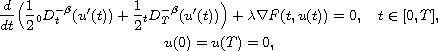where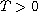,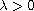is a parameter,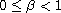,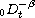and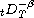are, respectively, the left and right Riemann-Liouville fractional integrals of order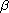,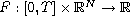is a given function. Our interest in the above system arises from studying the steady fractional advection dispersion equation. By applying variational methods, we obtain sufficient conditions under which the above equation has at least three solutions. Our results are new even for the special case when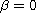. Examples are provided to illustrate the applicability of our results.

Submitted December 17, 2012. Published April 24, 2013.
Math Subject Classifications: 34B15, 34A08.
Key Words: Three solutions; fractional boundary value problem; variational methods.

Show me the PDF file (292 KB), TEX file, and other files for this article.Lingju Kong Department of Mathematics the University of Tennessee at Chattanooga Chattanooga, TN 37403, USA email: Lingju-Kong@utc.edu